# Gate Mock Test- 5: CS/IT

## 65 Questions MCQ Test GATE Computer Science Engineering(CSE) 2023 Mock Test Series | Gate Mock Test- 5: CS/IT

Description
Attempt Gate Mock Test- 5: CS/IT | 65 questions in 180 minutes | Mock test for GATE preparation | Free important questions MCQ to study GATE Computer Science Engineering(CSE) 2023 Mock Test Series for GATE Exam | Download free PDF with solutions
QUESTION: 1

### Choose the most approximate word from the options given below to complete the following sentence.If I had known that you were coming, I _______ you at the airport

Solution:

The sentence talks about a past incident and a condition has been mentioned. Thus option 2 fits here correctly. 'Would have' is the correct tense to be used here. Option 1 is illogical. 'Would' must be used here and not 'will' as it is in the past tense. Past perfect tense is incorrect here.

QUESTION: 2

### Choose the most approximate word from the options given below to complete the following sentence. I believe in the _____ of positive thinking thus always recommend these books to my clients.

Solution:

The word that fits here is a noun thus options 3 and 4 are eliminated. The form should be singular as for abstract nouns we do not use the plural form. Option 1 is thus the correct answer.

The word powerful is an adjective and 'empowering' is a verb.

QUESTION: 3

### Consider a circle of radius r. Fit the largest possible square inside it and the largest possible circle inside the square. What is the radius of the innermost circle?

Solution: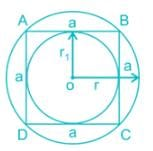The radius of outer circle = r

∴ Diagonal of square = 2r

Side of square (a)=√2r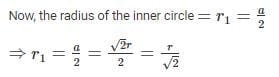QUESTION: 4

A bird files along the three sides of a field in the shape of an equilateral triangle at speeds of 3, 6, 8 km/hr respectively. The average speed of the bird is

Solution:

Average speed = Total distance/Total time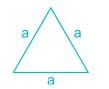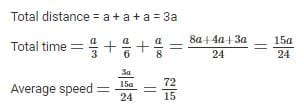QUESTION: 5

Choose the most appropriate words from the options given below to fill in the blanks.

She was ______ to travel abroad and _____ in the field of commerce as per her wishes.

Solution:

The sentence mentions a person doing something as per her wishes thus the first word must be a positive reaction. 'Restive' which means 'restless' and 'jinxed' which means 'cursed' are incorrect here. Between options 1 and 3,the word 'elated' which means 'too happy' and the word 'venture' which means 'undertake a risky or daring journey or course of action' fit here correctly. The word 'cease' means 'stop' and does not convey a proper meaning. Thus option 3 is the correct answer.

QUESTION: 6

In a 1600 m race around a circular track of length 400 m, the faster runner and the slowest runner meet at the end of the sixth minute, for the first time after the start of the race. All the runners maintain uniform speed throughout the race. If the faster runner runs at twice the speed of the slowest runner. Find the time taken by the faster runner to finish the race.

Solution:

As, the faster runner is twice as fast as the slowest runner, the faster runner would have complete two rounds by the time the slowest runner completes one round.

Their first meeting takes place after the fastest runner takes 6 min to complete two rounds and the slowest runner completes one round.

Time taken to complete one round by fastest runner = 6/2 = 3 minutes

Rounds needed to complete the race = 1600/400 = 4

Time taken to complete the race = 4 × 3 = 12 min

QUESTION: 7

Select the pair that best expresses a relationship similar to that expressed in the pair:

Horse: Foal

Solution:

The young one of a horse is called a foal. Similarly, the young one of a goose is known as gosling. Thus, option 2 contains the pair that best expresses a relationship similar to that expressed in the given pair. In all the other pairs, the first word expresses the male form of the latter.

QUESTION: 8

Read the following passage and find out the inference stated through passage.

Juvenile delinquency is also termed as Teenage Crime. Basically, juvenile delinquency refers to the crimes committed by minors. These crimes are committed by teenagers without any prior knowledge of how it affects the society. These kind of crimes are committed when children do not know much about outside world.

Which of the following Inferences is correct with respect to above passage?

Solution:

Since teenagers here have tender age and commit crimes unknown to its consequences, clearly they should not be treated as any criminal. They need to be taught so that they can make difference between right and wrong and do not commit these crimes in future. Hence inference in option 1 is correct.

Previous

QUESTION: 9

If n and y are positive integers and 450 y = n³, which of the following must be an integer?

Solution:

450 y = n³ implies that 450 y is the cube of an integer.

When we prime-factorize the cube of an integer, we get 3 (or a multiple of 3) of every prime factor.

8 is the cube of an integer because 8 = 2³ = 2*2*2.

Thus, when we prime-factorize 450 y, we need to get at least 3 of every prime factor.

Here's the prime factorization of 450 y:

450 y = 2 * 3² * 5² * y

Since 450 provides only one 2, two 3's, and two 5's, and we need at least 3 of every prime factor, the missing prime factors must be provided by y. Thus, y must provide at at least two more 2's, one more 3, and one more 5.

Smallest possible case:

y = 2² * 3 * 5

QUESTION: 10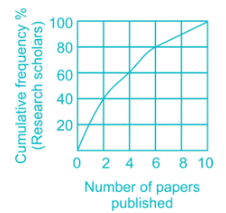The graph shows cumulative frequency % of research scholars and the number of papers published by them. Which of the following statements is true?

Solution:

In this problem, cumulative frequency is given, so converting into frequency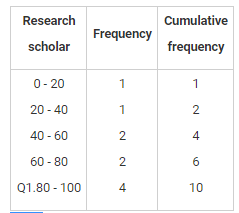From the table, it is clear that option b, i.e.

60% of the scholars published at least 2 papers is correct.

QUESTION: 11

Consider the following system of equations in three real variables x, y, z.

2x – 3y + 7z = 5

3x + y – 3z = 13

2x + 19y – 47z = 32

The system of equation has

Solution:

Augmented matrix will be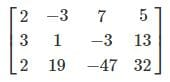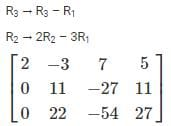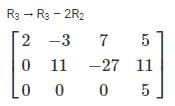Rank of A ≠ Rank of Augmented matrix

Hence given system of equations has no solution.

*Answer can only contain numeric values
QUESTION: 12

One of the addresses in a block is 135.16.27.116/20, the third octet (in decimal) of the first IP address in the block is _______.

Solution:

Concept:

Keep the n leftmost bits of given address in the block and set the 32 − n rightmost bits to 0s to ﬁnd the first address where n is the prefix length.

Calculation:

Here, prefix length is 20.

135.16.27.116 ⇒⇒ 10000111 00010000 00011011 01110100

The first 20 bits of this octal are fixed as 10000111 00010000 0001

The remaining 32 – 20 = 12 bits can get a maximum value as 0000 00000000.

So the possible first octal IP address is 10000111 00010000 00010000 00000000 which is 135.16.16.0

*Answer can only contain numeric values
QUESTION: 13

What is the output of the following C code:

int main( )

{

int p=8,q=-3,r=0;

int x,y,z;

x = p && q && r ;

y = p || q && r ;

z = p && q || r ;

printf ( "%d", x+y+z ) ;

return 0;

}

Solution: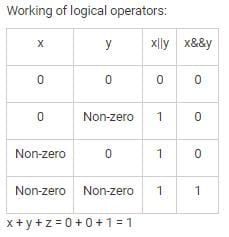QUESTION: 14

Consider two relational schemas:

emp(ID, name, address, phone number, deptID)

department(ID, managerID, deptname, location)

What does the following relational expression perform?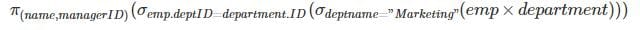Solution:

The expression retrieves name of all employees in the Marketing department along with their manager ID.

QUESTION: 15

Let q, r, and s represent “You can ride the roller coaster,” “You are under 4 feet tall,” and “You are older than 16 years old,” respectively. What is the logical expression for “You cannot ride the roller coaster if you are under 4 feet tall unless you are older than 16 years old"

Solution:
*Answer can only contain numeric values
QUESTION: 16

A continuous random variable, X is distributed in interval 0 – 10. The probability, P(x = 2) is ______

Solution:

In case of continuous random variable probability at a point = 0

QUESTION: 17

In a compiler, a lexical analyzer

Solution:

The lexical analyzer reads the stream of character making up the source program and groups the characters into meaningful sequences called as lexemes. For each lexeme, the lexical analyzer produces as output a token of the form <token name, attribute value>

*Answer can only contain numeric values
QUESTION: 18

Let L be the set of all strings generated by the regular automata over input alphabet {x,y}. The minimum number of states in a minimal DFA that accepts all the strings of length not more than 250 is ________.

Solution:

Strings of length not more than 250 means all those strings whose length is less than or equal to 250.

Note: If |w| <= n, the number of states in minimal DFA is n+2.

Therefore, the minimum number of states in a minimal DFA that accepts all the strings of length not more than 250 is 252.

*Answer can only contain numeric values
QUESTION: 19

A process executes the following code.

void main()

{

if(fork()==0)

{

for(int i = 0; i<5; i++)

{

printf(“Hello”);

}

}

else

for(int j = 0; j<5; j++)

{

printf(“Hello”);

}}

Find the number of times ‘Hello’ will be printed.

Solution:

fork() can be used to create a new process, known as a child process. This child is initially a copy of the parent but can be used to run a different branch of the program or even execute a completely different program. After forking, child and parent processes run in parallel. fork() returns 0 in child process and non-zero value in parent process.

QUESTION: 20

Bob wants people to know his public key but at the same time, he wants no one to accept a forged key as his. He decides to create a public key certificate. He goes to Certificate Authority(CA) where CA asks for Bob’s ______ key and signs the certificate with his _______ key.

Solution:

CA is a federal or state organization that binds a public key to an entity and issues a certificate. The CA has a well-known public key itself that cannot be forged. The CA checks Bob's identification. It then asks for Bob's public key and writes it on the certificate. To prevent the certificate itself from being forged, the CA signs the certificate with its private key.

*Answer can only contain numeric values
QUESTION: 21

Consider the following functions:

f1(a, b, c) = Ʃm(0, 1, 3, 5) + d(2, 4)

f2(a, b, c) = Ʃm(1, 6) + d(2, 3, 5)

How many functions are possible for f1 + f2?

Solution: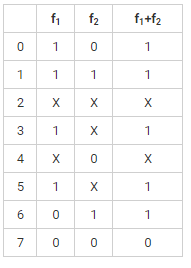Therefore, the number of functions possible = 22 = 4

*Answer can only contain numeric values
QUESTION: 22

Consider the statement

do

{

i = i + 1;

}

While(a[i] < b);

The minimum number of variables required in three address code of the above statement is _____.

Solution:

t1 = i + 1

i = t1

t2 = i * 8 // refers memory allocation for given data type

t3 = a[t2]

if t3 < b

Hence, 3 variables are required in three address code.

QUESTION: 23

Which of the following are one of the maximal and minimal elements respectively of the poset ({2, 4, 5, 10, 12, 20, 25}, /)

Solution: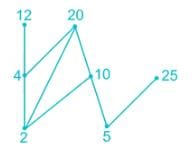Therefore, maximal elements are {12, 20, 25} and the minimal elements are {2, 5}.

QUESTION: 24

The post order traversal of a binary search tree is given by 25, 30, 20, 45, 60, 50, 40, 35. The pre order traversal of this tree will be given by:

Solution:

Post-order traversal: 25, 30, 20, 45, 60, 50, 40, 35

In order traversal is the arrangement of keys in ascending order. Therefore,

In order traversal: 20, 25, 30, 35, 40, 45, 50, 60

The tree is given by:

*Answer can only contain numeric values
QUESTION: 25

Consider the following weighted graph. Bellman-Ford algorithm is implemented on the given graph with source P. The shortest distance from source P to vertex T is ______.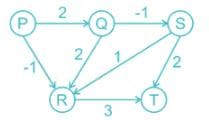Solution:
*Answer can only contain numeric values
QUESTION: 26

In a TCP connection, the client closes the connection using three-way handshaking. The client TCP sends the last segment an ack segment with an acknowledgment no. 2164. What is the value of sequence number in FIN + ACK segment sent by server TCP? Assume that there is no data transfer between client and server TCP.

Solution:

Concept:

The client TCP after receiving close command sends the first segment, FIN segment. In which only FIN flag is set.

Let the sequence no. be x and acknowledge no. be y.

The server TCP sends FIN + ACK segment after receiving the FIN segment.

Here, sequence no will be y and ack no will be x + 1.

The client TCP sends the last segment an ACK segment. This segment contains an acknowledgment number which is plus 1 the sequence number received in the FIN + ACK segment from the server.

Calculation:

Acknowledgment number in last segment i.e. ACK segment is 2164. Hence, sequence number in FIN + ACK segment is 2164 – 1 = 2163

QUESTION: 27

Consider the following languages:

I. {amb| m = 3n + 1, where m,n >=0}

II. {ap | p is a prime number}

III. {aibj | i ≠ 5j, where i,j>=0}

IV. {w | w Є {a,b}, na(w) = nb(w) + 1}

Which of the above languages are context free?

Solution:

I, III and IV are CFL because one comparison can be done by a push down automata at one time.

*Answer can only contain numeric values
QUESTION: 28

Consider a FAT-based file system which is stored on a disk of 900 GB. The data block size is 45000 bytes. The total overhead in each entry is 6 bytes in size. The maximum size of a file that can be stored on this disk is ______ MB

Solution:

Total number of entries = 2 ×× 107

Space occupied by overhead = 2 x 107 x 6 = 12 x 107

Maximum file size = total file system size – space consumed by overhead

= 90000 x 107 – 12 x 107

= 899880 MB

*Answer can only contain numeric values
QUESTION: 29

The chromatic number of a planar graph is not greater than ________

Solution:

The chromatic number of a planar graph is no greater than four.

QUESTION: 30

Consider the following grammar:

S→Pp

P→QR

Q→q|ε

R→r|ε

Find the value of first(P) and follow(Q)?

Solution:

First(S) = {p, q, r}

First(P) = {q, r, ε}

First(Q) = {q, ε}

First(R) = {r, ε}

Follow(S) = {\$}

Follow(P) = {p}

Follow(Q) = {p, r}

Follow(R) = {p}

QUESTION: 31

Consider the following C program:

#include <stdio.h>

void fun(int *p)

{

int i,j;

for(i = 0;i < 4; i++)

{

for(j=0;j<3;j++)

{

printf("%d\t", p[i]);

}

}

}

int main()

{

int a = {1, 5, 3};

int *p = a;

fun(p);

return 0;

}

What will be the output of the above program?

Solution:

The loop will be iterated for (4*3 = 12) times. Also, the remaining values of the array are initialized to 0.

Therefore, the output will have 12 terms and it will be: 111555333000

QUESTION: 32

What is the space complexity of insertion sort?

Solution:

Space Complexity of an algorithm is total space taken by the algorithm. The Insertion sort algorithm has to store all n inputs and using three more variables. So the space complexity of the algorithm is O(n). Auxiliary Space for insertion sort is O(1).

*Answer can only contain numeric values
QUESTION: 33

Consider direct mapping implementation of a cache of size 16 MB. The main memory has a capacity of 1 GB. Let the number of tag bits required is 'x' and the comparator latency is 20x ns. What is the hit latency?

Solution:

Line offset = Main memory size/ Cache size

= 230/224 = 26

Therefore, number of tag bits = 6

Hit latency = 20*6 = 120 ns

QUESTION: 34

Which of the following statements is false?

Solution:

Option 2 is incorrect.

Rollback causes the current transaction to be rolled back; that is, it undoes all the updates performed by the SQL statements in the transaction.Thus, the database state is restored to what it was before the first statement of the transaction was executed.

QUESTION: 35

In which of the following, framing bits are used?

Solution:

In synchronous TDM, synchronization between multiplexer and demultiplexer is a major issue. Therefore, one or more synchronization bits are usually added to the beginning of each frame. These bits are called as framing bits. It follows a pattern, frame to frame, that allows the demultiplexer to synchronize with the incoming stream so that it can separate the time slots accurately.

*Answer can only contain numeric values
QUESTION: 36

Th eigen vector of the matrix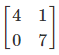are written in the form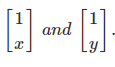.[1x]and[1y]. What is value of x + y?

Solution: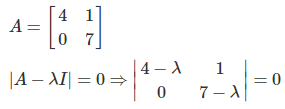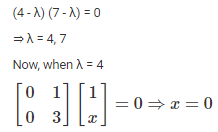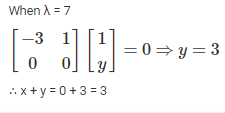QUESTION: 37

A finite automata over the input alphabet {x,y} is given below. What does the following deterministic finite automata perform?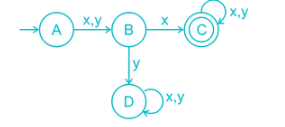Solution:

The language generated by the given automata is: {xx, yx, xxx, xxy, .........}

The given automata accepts all the strings whose second symbol from left hand side is x.

QUESTION: 38

In RSA cryptosystem, Bob wants to encrypt a message “RDX” using the values of 1 to 26 for letters A to Z. He uses p = 11, q = 19 and d = 103 to generate his public and private keys. What will be the cipher text generated by applying RSA algorithm (Encrypt each character individually)?

Solution:

p = 11

q = 19

ϕ(n)ϕ(n) = (p - 1)(q - 1) = 180

n = pq = 209

d e mod ϕ(n)ϕ(n) = 1

103 e mod 180 = 1

103 e = 180k + 1 …(k = 1,2,3,…)

e = 7

Ciphertext (C) = Mmod n

For ‘R’, M = 18

C = 187 mod 209

C = 9

For ‘D’, M = 04

C = 47 mod 209

C = 82

For ‘X’, M = 24

C = 247 mod 209

C = 73

QUESTION: 39

A company has three machines A, B and C. The machines produce 20%, 45% and 65% of identical goods respectively. Out of them the defective pieces produced by each machine are 0.01, 0.03, 0.15. What is the probability that a defective piece is selected and it is produced by machine ‘C’.

Solution: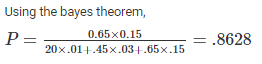QUESTION: 40

A BFS algorithm is implemented on the following graph. Which of the following nodes will be traversed at last if the root is ‘E’ and consider lexicographic ordering while traversing?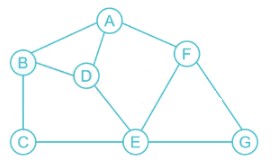Solution:

A queue is used to implement a breadth-first search.

Enque node E in the queue.

Queue: E

Mark it as visited. Dequeue Eand enqueue its non-visited adjacent nodes.

Queue: C D F G

Mark C as visited. Dequeue Cand enqueue its non-visited adjacent nodes.

Queue: D F G B

Mark D as visited. Dequeue Dand enqueue its non-visited adjacent nodes.

Queue: F G B A

Mark F as visited. Dequeue Fand enqueue its non-visited adjacent nodes.

Queue: G B A

Mark G as visited. Dequeue Gand enqueue its non-visited adjacent nodes.

Queue: B A

Mark B as visited. Dequeue Band enqueue its non-visited adjacent nodes.

Queue: A

Mark A as visited. Dequeue Aand enqueue its non-visited adjacent nodes.

Queue: Empty queue

*Answer can only contain numeric values
QUESTION: 41

What is the number of comparators required in an associative mapping implementation of cache where the size of main memory and the size of cache are 256 KB and 32 KB respectively? The block size if 512 bytes.

Solution:

Main memory size = 218 bytes

Cache size = 215 bytes

Block size = 2bytes

Number of cache lines = Cache size/Block size = 215/29 = 26

Therefore, number of comparators required = 26 = 64

*Answer can only contain numeric values
QUESTION: 42

Consider four processes P1, P2, P3, and P4. The arrival time and the burst time for each process are given in the table below. Find the maximum number of processes present in the waiting state if the operating system implements a shortest remaining time first scheduling algorithm?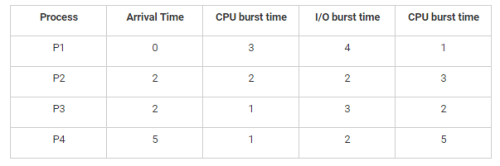Solution:

Concept:

In the ready state, the process is waiting to be assigned to a processor. In running state, instructions are being executed. While the processes are in running state it may ask for i/o thus the process goes into waiting state instead of the ready state.

Calculation:

Gantt chart for SRTF: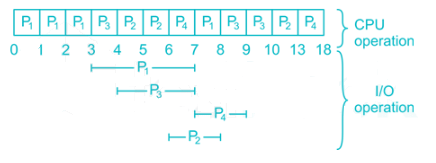*Answer can only contain numeric values
QUESTION: 43

The value of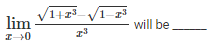Solution: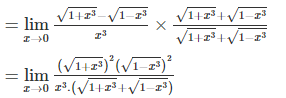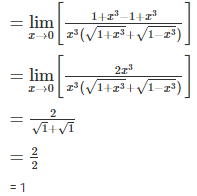QUESTION: 44

Construct the parsing table for the following LL1 grammar: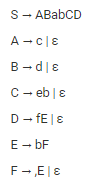Solution:

First(s) = {a, c, d}

First(A) = {c, ε}

First(B) = {d, ε}

First(C) = {e, ε}

First(D) = {f, ε}

First(E) = {b}

First(F) = {, , ε}

Follow(S) = {\$}

Follow(A) = {b, d}

Follow(B) = {a}

Follow(C) = {f, \$}

Follow(D) = {\$}

Follow(E) = {\$}

Follow(F) = {\$}

QUESTION: 45

In binary search tree, what happens to inorder successor when we delete a node that has both left and right child as non-empty?

Solution:

The correct answer is option 3 i.e. Inorder successor is either a leaf node or a node with empty left child.

Let X be the node to be deleted in a tree with root as ‘root’. There are three cases for deletion

1) X is a leaf node: We change left or right pointer of parent to NULL (depending upon whether X is left or right child of its parent) and we delete X

2) One child of X is empty: We copy values of non-empty child to X and delete the non-empty child

3) Both children of X are non-empty: In this case, we find inorder successor of X. Let the inorder successor be Y. We copy the contents of Y to X, and delete Y.

*Answer can only contain numeric values
QUESTION: 46

Consider an instruction pipeline in which a total of 5 cycles is required to complete the execution of the first instruction out of ‘n’ instructions. The speed up factor for the instruction execution without the pipeline compared to instruction pipeline is 3.75. Find the time (in nanoseconds) required to execute ‘n’ instructions without pipeline if maximum stage delay is 300 picoseconds.

Solution:

k = 5

Sk = 3.75

ττ = 300 picosecond = 0.3 ns

The time required to execute n instructions without pipeline = T1 = nkτnkτ

The time required to execute n instructions with pipeline = Tk = [k + (n – 1)]τ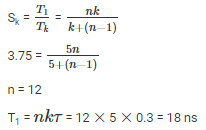*Answer can only contain numeric values
QUESTION: 47

Compute effective access time in seconds for a demand-paged memory. The memory access time is 180 ns and the average latency, seek time, transfer time is of 3 ms, 4ms, 1ms respectively. Assume the probability of page fault is 0.4.

Solution:

effective access time = (1 − p) x memory access + p x page fault time

effective access time = (1 − p) x memory access + p x (average latency + seek time + transfer time)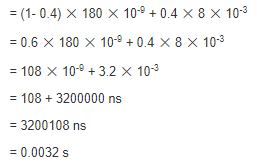QUESTION: 48

Consider the following schedules: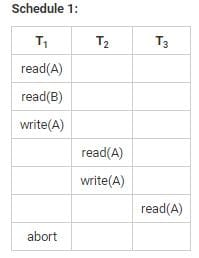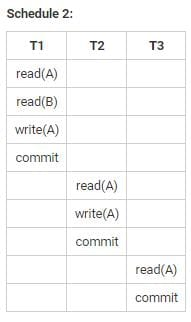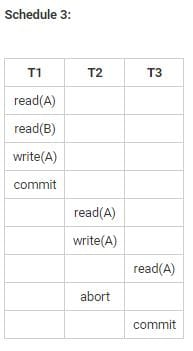Which of the above schedules are cascadeless schedules?

Solution:

Cascadeless schedule is one where, for each pair of transactions Ti and Tj such that Tj reads a data item previously written by Ti , the commit operation of Ti appears before the read operation of Tj.

Therefore, only Schedule 2 is the cascadeless schedule.

QUESTION: 49

Which of the following scheduling algorithms majorly suffer from starvation?

Solution:

A process that is ready to run but waiting for the CPU can be considered blocked. A priority scheduling algorithm can leave some low- priority processes waiting indefinitely. In a heavily loaded computer system, a steady stream of higher-priority processes can prevent a low-priority process from ever getting the CPU.

QUESTION: 50

Consider the grammar G =({A, B}, {x,y}, P, A}, where P is given by:

A → xA/yB/y

B → xB/yB/x/y

What is the language generated by the given grammar?

Solution:

The language generated by the given grammar is x*y(x+y)*.

The DFA for the given language is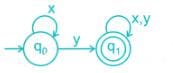*Answer can only contain numeric values
QUESTION: 51

The least number of temporary variables required to create a three-address code in static single assignment form for the expression p+q*r-s/(q*r)

Solution:

t1= q*r

t2= p+t1

t3= q*r

t4= s/t3

t5= t2-t4

Hence, minimum 5 variables are required.

QUESTION: 52

Which of the following statements is false?

Solution:

Sometimes the removal from a graph of a vertex and all incident edges produces a subgraph with more connected components. Such vertices are called cut vertices(or articulation points). The removal of a cut vertex from a connected graph produces a subgraph that is not connected.

QUESTION: 53

Consider the following memory values and a single address machine with an accumulator.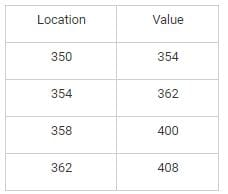Which of the following instructions will load 408 into accumulator?​

Solution:

Indirect addressing mode refers to the address field refer to the address of a word in memory.

Above instruction points to memory location 354 which contains 362​

Above instruction points to memory location 362 which contains 408​

Above instruction points to memory location 362 which contains 408

QUESTION: 54

Which of the following is equivalent to the expression xy+x′z+yz ?

Solution:

The expression is equivalent to xy+x′zxy+x′z.

Proof: xy+x′z+yz

xy+x′z+yz(1)

xy+x′z+yz(x+x′)

xy+x′z+xyz+x′yz

xy(1+z)+x′z(1+y)

xy+x′z

QUESTION: 55

Consider the recurrence relation:

T(n) = 16T(n/4)+n!

Above recurrence relation represents the running time of an algorithm. Which of the following represents the time complexity of the algorithm?

Solution:

On comparing with equation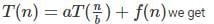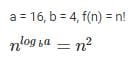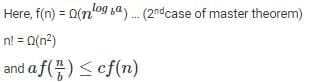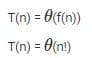QUESTION: 56

What should be substituted in the place of (A) to find the maximum element out of the two elements?

int main ()

int a = 100, b = 200;

int *p = &a;

int *q = &b;

(A)

return 0;

}

Solution:

Option 1 can be used to find the maximum element of the two elements.

*Answer can only contain numeric values
QUESTION: 57

Consider the grammar with the following translation rules and X as the start symbol.

X -> X1* Y      {X.value = X1.value * Y.value}

X -> Y              {X.value = Y.value}

Y -> Y1- Z       {Y.value = Y1.value - Z.value}

Y -> Z              {Y.value = Z.value}

Z -> digit          {Z.value = digit.value}

What is the value for the root of the parse tree for the expression: 54 – 5 * 1 * 7 – 4?

Solution:

In above grammar – has higher precedence order than *.

Hence, - will be evaluated first.

(54 – 5) * 1 * (7 – 4)

49 * 1 * 3

147

QUESTION: 58

The following C function inserts a new element after a specific element in a doubly linked list.

struct Node {

int data;

struct Node* next;

struct Node* prev;

};

void insertAfter(struct Node* previous, int value)

{

struct Node* element = (struct Node*)malloc(sizeof(struct Node));

element->data = value;

element->next = previous->next;

previous->next = element;

element->prev = previous;

if (element->next != NULL)

__________

}

Choose the correct alternative to replace the blank line.

Solution:

Allocate a new node. Assign value to new node.

element->data = value;

Make next of new node as next of previous.

element->next = previous->next

Make the next of previous as new node i.e. element

previous->next = element;

Make previous as previous of new node

element->prev = previous

Make new node as next node of previous

element->next->prev = element;

QUESTION: 59

In the question below, a Turing machine M over  {0,1} is given. Which of the following statements is true about M?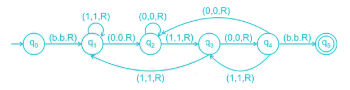Solution:

The given Turing machine reads all the 1's and 0's and keeps moving right. It enters the loop at state q4 and iterates until it encounters a blank. The loop has a pattern 010 and the machine accepts the string which ends with 010.

Therefore, the machine M accepts the set of all strings over {0,1} ending with 010.

QUESTION: 60

Find the time complexity of the following C code if n > m.

int foo(int m, int n)

{

if(m>n)

swap(n,m);

if(n%m == 0)

return m;

else

return foo(m, n%m);

}

Solution:

At each recursive step one of the arguments will reduce by half (almost). Here n > m hence time complexity is O(log n).

QUESTION: 61

The generating function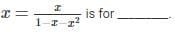Solution:

The fibonacci series is <0,1,1,2,3,5,8....>

The generating function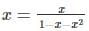x=x1−x−x2 is for fibonacci series.

*Answer can only contain numeric values
QUESTION: 62

Consider the following C program:

#include

int fun(char *s, char *t)

{

for(; *s == *t; s++, t++)

if(*s == '\0')

return 0;

return *s - *t;

}

int main()

{

char a="Hello";

char b="Help";

char *p = a;

char *q = b;

int x=fun(p,q);

printf("%d", x);

return 0;

}

What will be the output of the program?

Solution:

The above C program is used to compare two strings and return an integer. If the first character of two strings are equal, next character of two strings are compared. This continues until the corresponding characters of two strings are different or a null character '\0' is reached.

In the given C program, the first unmatched character between the two strings a and b is the fourth character. The ASCII value of 'l' is 108 and that of 'p' is 112.

Therefore, the output will be 108-112 = -4.

*Answer can only contain numeric values
QUESTION: 63

A sender uses a Stop-and-Wait protocol for transmission of 8000 k-bits size frames on a 1Gbps satellite channel with a propagation delay of 400 ms. What will be the link utilization(%) if a probability of single frame error is 0.001?

Solution:

Frame size (L) = 8 x 106 bits

Bandwidth (B) = 1 x 109 bps

Propagation delay = Tp = 400 ms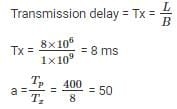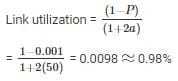QUESTION: 64

Consider a system with three resource types and the vector Available initialized to (5,3,2). If process P0 asks for (2,1,1), it gets them. If P1 asks for (2, 2,1), it gets them. Then, if P0 asks for (0,0,1), it is blocked (resource not available). If P2 now asks for (2,0,0), it gets the available one (1,0,0) and one that was allocated to P0 (since P0 is blocked). P0’s Allocation vector goes down to (1,1,1), and its Need vector goes up to (1,0,1). Which of the following statements is TRUE about the given system?

Solution:

Deadlock cannot occur because preemption exists. A process may never acquire all the resources it needs if they are continuously preempted by a series of requests such as those of process P2.

*Answer can only contain numeric values
QUESTION: 65

A counting semaphore was initialized to 17. Then 8P (wait) operations and ‘n’V (signal) operations were completed on this semaphore. Find the value of n if total 14 down operations can be carried out successfully after 8 wait operation and n signal operations?

Solution:

Initial value of semaphore = 17

After each wait operation, semaphore value decremented by 1.

8P(wait or down) operations were completed

Semaphore value = 17 - 8 = 9

After each signal operation, semaphore value incremented by 1.

5V(signal or up) operations were completed and resulting value of the semaphore is 14

Semaphore value = 9 + n = 14

which means total 5 signal operations were completed.Use Code STAYHOME200 and get INR 200 additional OFF Use Coupon Code